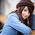## Monday, 26 June 2017

### Find the next smallest palindrome for a number

For a given a number, find the next smallest palindrome larger than this number.

Examples:
1. If the input number is “3 4 6 5 6”, the output should be “3 4 7 4 3”.
2. If the input number is “9 9 9 9”, the output should be “1 0 0 0 1”.

Approach:
There can be three different cases those should be handled separately.
1) The input number is palindrome and has all 9s.
Example
Input: 9 9 9 9
Output:1 0 0 0 1.

It can be get by adding 2 into the number.

2) The input number is not palindrome.
Example
Input: 2 3 4 5
Output:2 4 4 2.

3) The input number is palindrome and doesn’t have all 9s.
Example
Input: 2 3 3 2.
Output:2 4 4 2.

package com.geeks;

public class NextGreaterPalindrome {

/**
* getNextPalindrome
* @param number
* @author rajesh.dixit
* @return
*/
private static void getNextPalindrome(int number) {

/** To handle the case when all digits in number are 9. */
if((int)Math.log10(number+1)!=(int)Math.log10(number)) {
System.out.println("All 9s");
System.out.println("Next Palidrome for the number "+ number +" is: "+(number+2));
}

int[] array = getNumberArray(number);

int length = array.length;

// find the index of middle digit
int mid = length/2;

// A boolean variable to check if copy of left side to right is sufficient or not
boolean leftSmaller = false;

// end of left side is always 'mid -1'
int i = mid - 1;

// Beginning of right side depends if n is odd or even
int j = (length%2)!=0? mid + 1 : mid;

while(i >= 0 && array[i]==array[j]) {
i--;j++;
}

/*
* leftSmaller when the left side array small then right one
* or left array is equal to right array (i<0)
* */
if(i<0 || array[i]<array[j]) {
leftSmaller = true;
}

// Copy the mirror of left to right
if(!leftSmaller) {
while (i >= 0) {
array[j] = array[i];
j++;
i--;
}
} else {
/* Case: When left side is small or equal to right side.
* need to increment the middle element.
* */
i = mid - 1;
int carry = 1;
if(length%2==1) {
int temp = array[mid]+carry;
carry = temp/10;
array[mid] = temp%10;
j = mid+1;
} else {
j = mid;
}

while(i>=0) {
int temp = array[i]+carry;
carry = temp/10;
temp = temp%10;
array[j] = temp;
array[i]  = temp;
i--;j++;
}
}

System.out.print("Next Palidrome for the number "+ number +" is: ");
printArray(array);
}

/**
* Convert number into int Array
* @param number
* @return int[] array
*/
private static int[] getNumberArray(int number) {

int length = (int)(Math.log10(number)+1);

int[] array = new int[length];

for (int i = 0; i < length; i++) {
int val = number%10;
number = number/10;
array[length-i-1] = val;
}
return array;
}

/**
* Utility Method to print numbers.
* @param number
*/
private static void printArray(int[] number) {
for(int value : number) {
System.out.print(value);
}
}

/**
* Driver Method
* @param args
*/
public static void main(String[] args) {

int number = 34656;
getNextPalindrome(number);
}
}

1.2.3.